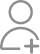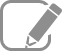Take Class 11 Tuition from the Best Tutors

•Affordable fees
•1-1 or Group class
•Flexible Timings
•Verified Tutors

Search in

# Calculate the degree of ionization of 0.05M acetic acid if its pKa value is 4.74. How is the degree of dissociation affected when its solution also contains (a) 0.01M (b) 0.1M in HCl ?Follow 1AnswerWhen HCl is added to the solution, the concentration of H+ ions will increase. Therefore, the equilibrium will shift in the backward direction i.e., dissociation of acetic acid will decrease. Case I: When 0.01 M HCl is taken. Let x be the amount of acetic acid dissociated after the addition of HCl. As...When HCl is added to the solution, the concentration of H+ ions will increase. Therefore, the equilibrium will shift in the backward direction i.e., dissociation of acetic acid will decrease.

Case I: When 0.01 M HCl is taken.

Let x be the amount of acetic acid dissociated after the addition of HCl.As the dissociation of a very small amount of acetic acid will take place, the values i.e., 0.05 – x and 0.01 + x can be taken as 0.05 and 0.01 respectively.Case II: When 0.1 M HCl is taken.

Let the amount of acetic acid dissociated in this case be X. As we have done in the first case, the concentrations of various species involved in the reaction are:Now ask question in any of the 1000+ Categories, and get Answers from Tutors and Trainers on UrbanPro.com

Find Class 11 Tuition near you

Looking for Class 11 Tuition ?

Learn from the Best Tutors on UrbanPro

Are you a Tutor or Training Institute?

Join UrbanPro Today to find students near you
X

### Looking for Class 11 Tuition Classes?

The best tutors for Class 11 Tuition Classes are on UrbanPro

• Select the best Tutor
• Book & Attend a Free Demo
• Pay and start Learning### Take Class 11 Tuition with the Best Tutors

The best Tutors for Class 11 Tuition Classes are on UrbanPro